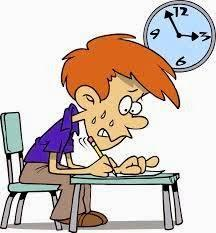# Basic Of Civil Engineering Lab Exam

10 Questions | Total Attempts: 108SettingsIndira Gandhi Institute of Technology, Sarang Metallurgy and Materials Science Engineering Electronics and Telecommunication Engineering Steps For Accessing Your Exam Online: Close all programs, including email Click on the https://www. Proprofs. Com/quiz-school/preview. Php? Title=mjq0ntuyoaawri to open the exam link provided in the email from The College. Click Logon To Exam at the bottom of the screen. Have your Proctor enter the Username and Password provided in the email from The Coll

• 1.
What type of force induced in truss?
• A.

Compressive force

• B.

Tensile force

• C.

Both a. and b.

• D.

None of the above

• 2.
Find the force in member CE?
• A.

5.57KN

• B.

6.63KN

• C.

0KN

• D.

9.93KN

• 3.
An object of mass m is placed on a frictionless inclined plane as indicated below. Which of the following expressions represent the normal force on the mass due to the inclined plane?
• A.

Mg tan θ

• B.

Mg

• C.

Mg cos θ

• D.

Mg sin θ

• 4.
Which apparatus used to measure the specific gravity of the bottle?
• A.

• B.

Pycnometer

• C.

Specific gravity bottle

• D.

Bucket

• 5.
The standard dimension of the Brick?
• A.

215 mm x102.5 mm x 65 mm

• B.

200mmx103mm x 65 mm

• C.

102mm102.5 mm x 65 mm

• D.

90mmx102.5 mm x 65 mm

• 6.
A sphere weighing 1000 N is supported by two wooden plates as shown in figure. Evaluate the reactions at the points A and C.
• A.

866.03 N,500 N

• B.

503.23N,603.33N

• C.

650N,300N

• D.

200N,320N

• 7.
Specific gravity of cement comes around?
• A.

5.03

• B.

6.23

• C.

3.15

• D.

8.23

• 8.
Determine the y coordinate of centroid of the area in the shape of circle as shown.
• A.

4R/π

• B.

2/π

• C.

2R/3

• D.

2R/5

• 9.
The value of Normal reaction (R) for the following figure is
• A.

W – PSinθ

• B.

W + PSinθ

• C.

P + WSinθ

• D.

P – WSinθ

• 10.
Turbidity measured in which scale?
• A.

Platinum

• B.

Cobalt

• C.

Silica

• D.

NickelBack to top